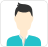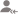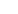truePost a Lesson

Answered on 04 May CBSE/Class 10/Mathematics Tuition/Class IX-X Tuition Ratio and ProportionSai Divya Nedunuri

MBA Finance Graduate and a Semi chartered accountant

0.75/x = 5/8 By cross multiplying, we get 5x = 0.75*8 Therefore, x= 6/5 = 1.2
Dislike Bookmark

Answered on 19/11/2016 CBSE/Class 10/Mathematics Tuition/Class IX-X Tuition Ratio and ProportionRicha Kumari

-1
Dislike Bookmark

Answered on 16/04/2019 CBSE/Class 10/Mathematics Tuition/Class IX-X Tuition Ratio and ProportionJanhavi.singh

49:9
Dislike Bookmark

Answered on 05/12/2016 CBSE/Class 10/Mathematics Tuition/Class IX-X Tuition Ratio and ProportionChandan Singh

Tutor

Mean proportion = square root of (25*64) = 40 Ans.
Dislike Bookmark

Answered on 22/11/2016 CBSE/Class 10/Mathematics Tuition/Class IX-X Tuition Ratio and ProportionRAM MOHAN A.

TEACHING IS MY PASSION-I STILL STRIVE TO BETTER MY TEACHING ABILITIES

The answer is 5:1. SOL :- x : y=9 : 10==> 10 x=9 y==> 5 x = 9 y/2, substituting 9y/2 for 5x in ( 5 x + 3 y) : (5 x - 3 y) gives 5: 1
Dislike Bookmark

Answered on 25/11/2016 CBSE/Class 10/Mathematics Tuition/Class IX-X Tuition Ratio and ProportionNagesh Guledagudda

Programming Passionate

(B/C ) * (C/D)= (6/7) * (14/15) => B/D = 4/5 => (A/B)*(B/D)=(4/5)*(4/5) => A/D = 16/25.
Dislike Bookmark

Answered on 20/03/2018 CBSE/Class 10/Mathematics Tuition/Class IX-X Tuition Ratio and ProportionVikas Verma

Tutor

4 : 5.
Dislike Bookmark

Answered on 06/12/2016 CBSE/Class 10/Mathematics Tuition/Class IX-X Tuition Ratio and ProportionSarvajeet Kumar

An Experienced Trainer

(By Componendo and Dividendo) 8a:18b=8c:18d or a:b = c:d.
Dislike Bookmark

Answered on 08/12/2016 CBSE/Class 10/Mathematics Tuition/Class IX-X Tuition Ratio and ProportionRama Krishna Ravulapalli

Rs. 1,90,085.
Dislike Bookmark

Answered on 07/12/2016 CBSE/Class 10/Mathematics Tuition/Class IX-X Tuition Ratio and Proportion

If x : y : z, show that x : z = (square of x) : (square of y)?Shambhavi S.

As we know that , x:y:z , this implies that, y^2=x*z. Simplifying x:z=x^2:y^2, we get, y^2=x*z, which is the same as above. Thus, they are equal.
Dislike Bookmark

UrbanPro.com helps you to connect with the best in India. Post Your Requirement today and get connected.

Overview

Questions 15

Total Shares50 Followers

## Top Contributors

Connect with Expert Tutors & Institutes for Ratio and Proportion

x

X

### Find Tutors, Trainers & Institutes near you

Post requirement and connect with the tutors in your locality

• Post a learning requirement
• Get customized responses
• Compare and select the best### Want to learn something New?

Find best tutors, trainers & institutes near you on UrbanProUrbanPro.com is India's largest network of most trusted tutors and institutes. Over 55 lakh students rely on UrbanPro.com, to fulfill their learning requirements across 1,000+ categories. Using UrbanPro.com, parents, and students can compare multiple Tutors and Institutes and choose the one that best suits their requirements. More than 7.5 lakh verified Tutors and Institutes are helping millions of students every day and growing their tutoring business on UrbanPro.com. Whether you are looking for a tutor to learn mathematics, a German language trainer to brush up your German language skills or an institute to upgrade your IT skills, we have got the best selection of Tutors and Training Institutes for you. Read more